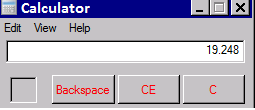# Decimal Spaces

Jump to: navigation, search

You can increase the number of digits after the decimal place in SME for Non-Currency fields up to 6 digits by going to Setup module > Company > Systems. Locate the Number of digits after the decimal for Non-Currency field and enter the number. Currency decimal places are handled by the Windows operating system and are set as outlined below. (Note: Decimals are rounded based on Banker/Math rules and will round up for fields requiring two decimal places and for QuickBooks).

SME is programed to use the SQL money datatype for all currency fields. This datatype is limited to four(4) places after the decimal. In calculations that exceed the 4th place, and use the 5th place, the 5th place value is not used. E.G. .34175 – would be stored as .3417. (Note that the 5th place, is not rounded, it’s not used by SQL). This may result in a difference if your division of the cost exceeds 4 places. The functionality behind this is due to syncing with QB as QB sums the total and then rounds, as does SME, and Excel, calculators, etc., round each value individually and then sum. The result is that there may be cases where the calculated cost will be a penny off. We’ve investigated modifying the data type however we have not due to the issues that raises with QB.

If the currency decimals don't change in SME, you need to change your settings in Windows.
1) In WIndows, open the Control Panel
2) Double click on Regional and Language Options
3) On the format tab, click Customize this format
4) In the customize Regional Options window, click the Currency tab and set the number of decimal spaces in the No. of digits after decimal field.
5) Click Apply and Ok

The items are summed and then rounded. A calculator does rounding by first rounding the individual amounts and then totals the sum. Ex:

Excel Rounding: At 2 places: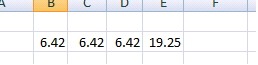Note that the total = 19.25 Expanded to 3 places: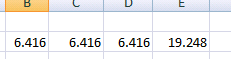SME/QB rounding: 6.416 + 6.416 + 6.416 = 19.25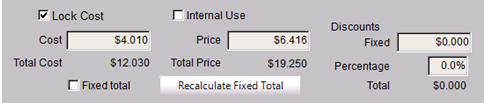Totaled price first: 6.146 * 3 = 19.248 – or 19.25
QuickBooks Total at 3 places: 6.416 = 19.25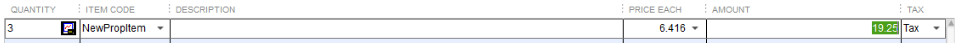MS Calculator rounding at 3 places: 6.416 (rounded = 6.42) * 3 = 19.26